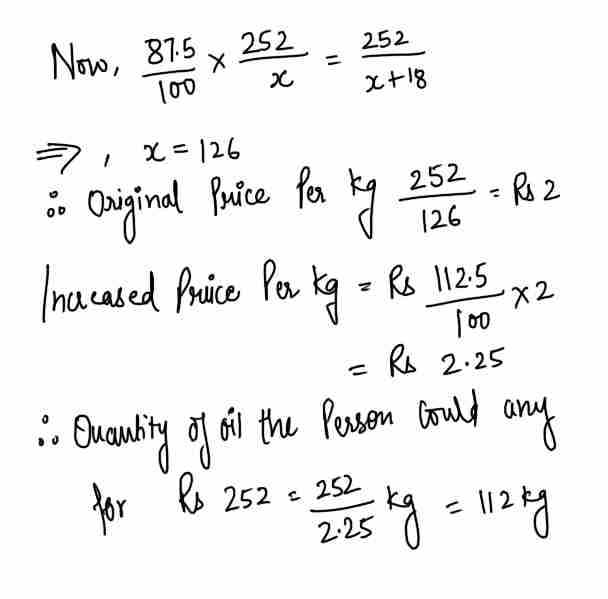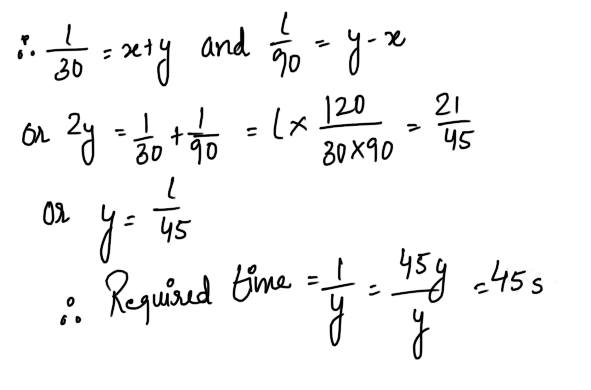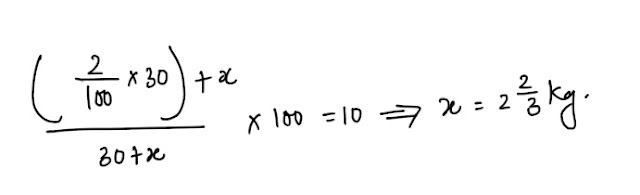# Mixed Aptitude Quiz - 13 (For SBI Clerical 2016)#### Ques 1.

If the price of oil falls by 12 1/2 % , a person can buy 18 kg more of oil for Rs 252 than before.If the price had risen by 12 1/2 % , how much oil could he have bought for the same sum ?

(a) 112 kg
(b) 110 kg
(c) 120 kg
(d) 125 kg
Ans 1. suppose that the person buys x kg of oil for Rs 252 before the fall in price.After the fall in price he can buy x + 18 kg of oil for Rs 252.#### Ques 2.

How many numbers between 100 and 300 begin or end with 2 ?
(a) 20
(b) 40
(c) 110
(d) 180
Ans 2.All the numbers from 200 to 299 with 2. Thus, there are 100 such numbers.In addition to this , numbers like 102, 112, 122, …., 192 end with 2. There are 10 such numbers.
Required number = 100 + 10 = 110

#### Ques 3.

If the sides of a triangle be in the ratio 2 : 3 : 4 , find the ratio of the corresponding altitudes,
(a) 3 : 2: 1
(b) 6: 4 : 3
(c) 4: 5 : 6
(d) None of these
Ans 3. Let the corresponding altitudes be h1, h2, and h3,
Now area of the triangle taking the different sides bases separately would be the same .#### Ques 4.

The sum of all two -digit numbers which are multiple of 3 is
(a) 1665
(b) 1500
(c) 2125
(d) None of these
Ans 4. The required sum is : 12 + 15 + 18 +……+ 99 which Is the sum of an A.P. whose first term is 12 ,last term is 99 and common difference is 3.
. . 12 + ( n -1) 3 = 99 => n = 30
Hence, the sum is : 30/2 ( 12 + 99) = 1665.

#### Ques 5.

John bought five mangoes and ten oranges together for forty rupees. Subsequently, he returned one mango and got two oranges in exchange .The price of an orange would be
(a) Rs 1
(b) Rs 2
(c) Rs 3
(d) Rs 4
Ans5. Let x be the price of a mango and y be the price of an orange.
5x + 10 y = 40; x = 2y
. . 10y + 10y= 40 or y =2
Hence, the price of an orange.

#### Ques 6.

Three machines A, B and C can be used to produce a product. Machine A will take 60 hours to produce a million units. Machine B is twice as fast as Machine A . Machine C will take the same amount of time to produce a million units as A and B running together.How much time will be required to produce a million units if all the three machines are used simultaneously ?
(a) 12 hr
(b) 10 hr
(c) 8 hr
(d) 6 hr
Ans 6. The rates at which the three machine A, B, and C work are in the ratio 1 : 2 : 3.
If A can can produce 1 million units in 60 hours, A , B and C together can produce the same number of units in 60 / 1 + 2+ 3 i.e. 10 hours.

#### Ques 7.

The half -yearly divided on 250 shares of Rs 12.50 each at 8 % p.a. will be
(a) Rs 125
(b) Rs 225
(c) Rs 120
(d) Rs 126
Ans 7.#### Ques 8.

A man can walk up a moving "up" escalator in 30 seconds .The same man can walk down this moving "up" escalator in 90 seconds .Assume that his walking speed is same upwards and downwards .How much time will he take to walk up the escalator , when it is not moving ?
(a) 30 s
(b) 45 s
(c) 60 s
(d) 90 s
Ans 8. Let the length the man covers be i.Let the speed of the escalator be x and the speed of the man be y.#### Ques 9.

Let n be any positive integer.Then n3-n is divisbile
(a) always by 12
(b) never by 12
(c) always by 6
(d) never by 6
Ans 10. N3 – n = ( n – 1) n( n + 1)
Now, (n -1) , n ( n+1) are there consecutive integers, Therefore , one of them should be a multiple of 3 and one at least out of them should be even .Hence, the product ( n – 1) n ( n + 1) should be a multiple of 6.

#### Ques 10.

Hoe many kilograms of pure salt must be added to 30 kg pf a 2 % solution of salt and water to increase it to a 10% solution ?
(a) 3 kg
(b) 15 kg
(c) 2 2/3 kg
(d) 14 1/3 kg
Ans 10.#### Ques 11.

The smallest number which when divided by 4, 6 or 7 leaves a remainder of 2 , is
(a) 44
(b) 62
(c) 80
(d) 86
Ans 11. L.C.M. of 4,6, 7, is 84.
Hence, the required number is 84 + 2 i.e. 86.

#### Ques 12.

If a sum amounts to Rs 960 in two years at simple interest of 10% p.a., what will be the compound interest on the same amount in the same period at the same rate of interest ?
(a) Rs 158
(b) Rs 166
(c) Rs 168
(d) Rs 148
Ans 12.